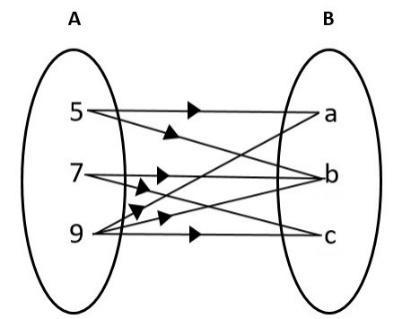Courses
Courses for Kids
Free study material
Free LIVE classes
More# Write the relation, represented by the arrow diagram given below, in roster form. Also write the domain and range of the relation.Verified
325.5k+ views
Hint: Here we have to write the relations according to the arrows represented from set A to set B in roster form which means we have to write the relations listed in braces.

In the figure given above, there are two sets A and B, where A contains the elements 5, 7, 9 and B contains the elements a, b, c.
Let R be the relation from A to B.
We know that there are three ways to represent the sets. They are
Roster form
Set builder form
Venn diagram form
We also know that representing the sets in roster form means, writing all the elements of the sets listed in braces.
Here we can represent the sets A and B in Roster form. i.e. the sets A and B can be written as follows:
A = {5, 7, 9}
B = {a, b, c}
In the arrow diagram given above, the relation between the sets are clearly given. Hence from the diagram we can say that 5 is related to a and b, 7 is related to b and c and 9 is related to a, b and c.
Therefore, we can represent the relation in roster form as:
R = {(5, a), (5, b), (7, b), (7, c), (9, a), (9, b), (9, c)}
Next, we have to identify the domain and range of the relation.
Here, domain is the set of independent values and range is the set of dependent values.
Here, the independent set is A, therefore the domain is A.
Domain = {5, 7, 9}
Similarly, the dependent set here is the set B. i.e. the range is B.
Range = {a, b, c}

Note: When you represent the relation in roster form from an arrow diagram, look at the arrow and write the relation. Here, the relation is from A to B, therefore you have to write the first element from the set A and the second element from the set B. Don’t interchange, it may lead to wrong answers.
Last updated date: 29th May 2023
Total views: 325.5k
Views today: 8.83k